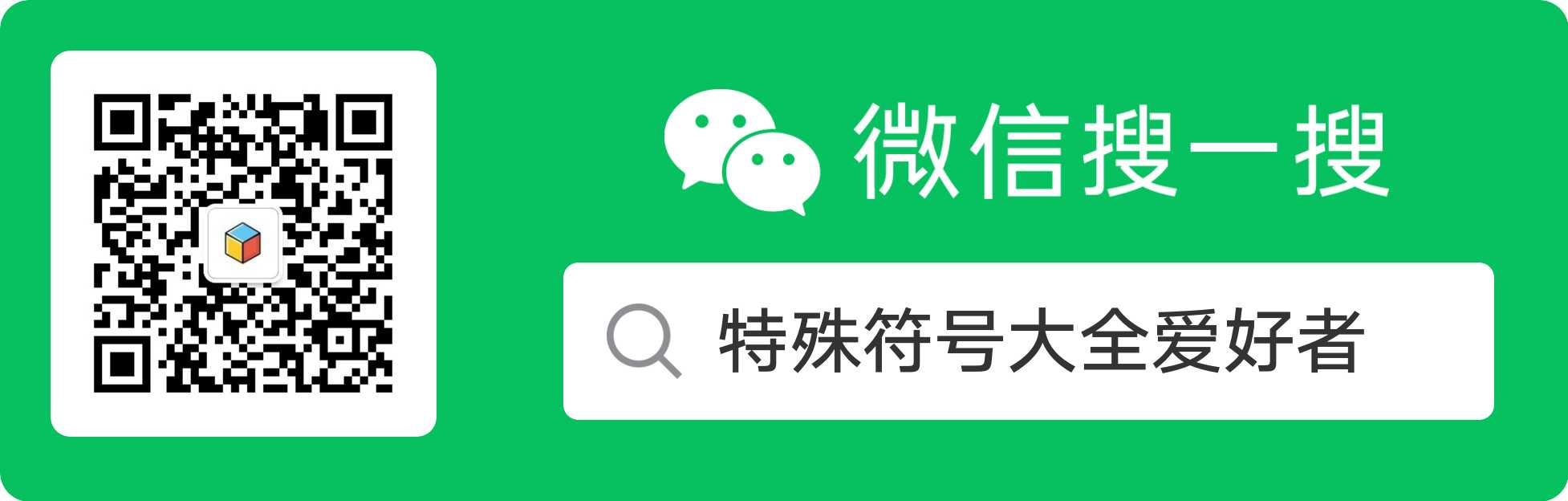# 动物颜文字表情符号

U•ェ•*U 狗狗

（￣。。￣） 河马

(°°)～　(°°)～　(°°)～ 蝌蚪

m( =∩王∩= )m 老虎

o(=•ェ•=)m 猫

ヽ(=^･ω･^=)丿 高兴猫

~o( =∩ω∩= )m 喵

≡ω≡ 喵呜

o( =•ω•= )m 喵星人

--(˙<>˙)/--  鸟

( ఠൠఠ )ﾉ  牛

_(:驴」∠)_ 躺枪驴

_(:马」∠)_ 躺枪马

(+(工)+╬) 熊

(*￣(エ)￣) 熊

(￣(●●)￣) 猪

(:◎)≡ 章鱼

(..)nnn 毛毛虫

≡[。。]≡ 螃蟹

<□:≡ 乌贼

<。)#)))≦ 烤鱼